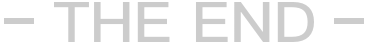preg_match_all("/src=["|'|s]{0,}(http://([^>]*).(gif|jpg|png))/isU",\$body,\$img_array);
\$img_array = array_unique(\$img_array);

 ```preg_match_all("/src=["|'|s]{0,}(http://([^>]*).(gif|jpg|png))/isU",\$body,\$img_array); preg_match_all("/src=["|'|s]{0,}(https://([^>]*).(gif|jpg|png))/isU",\$body,\$img_array_https); \$img_array = array_unique(\$img_array); \$img_array_https = array_unique(\$img_array_https); \$img_array=array_merge_recursive(\$img_array,\$img_array_https);```

 ```if(!preg_match("#^http://#i", \$value)) { continue; } ```

 ```if(!preg_match("#^http://#i", \$value)&&!preg_match("#^https://#i", \$value)) { continue; } ```